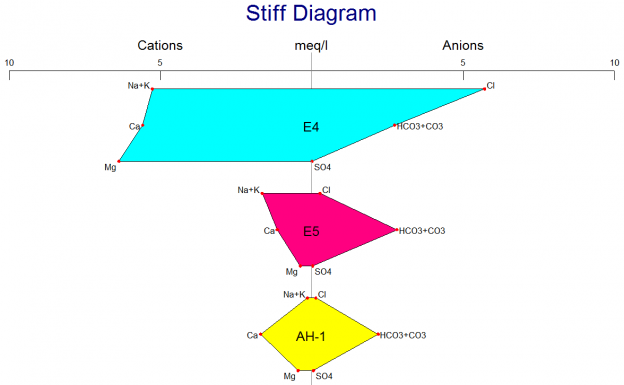# RockWorks Hydrochemistry, Hydrology Diagrams

Use RockWorks to create a variety of hydrological and hydrochemical diagrams.

Piper diagrams

Piper diagrams illustrate ion concentrations for multiple samples. Include Total Dissolved Solids circles and scale bar, uniform or custom symbols for each sample, sample legend.

Stiff Diagrams

Stiff diagrams illustrate relative ion concentrations for multiple samples. Include one to many polygons in a single diagram. Label polygons with sample number, define polygon fill options and color.

Stiff Diagram Maps

• Display relative ion concentrations for multiple samples on a map
• Many scaling options
• Options for labels and axes
• Great for showing water chemistry variations

Durov Diagrams

Plot hydrochemistry data as Durov diagrams, with optional point density of TDS color contours.

Other Hydrochemistry Tools

• TDS (Total Dissolved Solids) calculations
• Ion balance calculations

Well Drawdown

• Compute drawdown for a single well using the Theis equation and display as a report or diagram
• Compute drawdown for multiple pumping or injection wells listed in data sheet (also using Theis equation), display as 2D or 3D potentiometric surface map